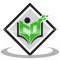## Dynamics Rotational MotionUpdated on 30-Jan-2023 18:06:03
Introduction There can be many types of motion exerted by a body like translational motion, rotational motion, circular motion, uniform motion, etc. In rotational motion, rigid bodies which have a definite size and definite shape are considered. The particles present in a body about a fixed axis move in a circular path. So to understand the concept of rotational motion you must know about circular motion. Here the terms are included in angular form. What is Rotational Motion? If a force is applied to any object at the corner side of it(not on the centre of mass), then we see ... Read More

## PulleyUpdated on 13-Oct-2022 11:19:47
Introduction Pulleys are often denoted as the simple machines that are used to carry or lift a heavy load in construction areas or even in sets designing. Pulleys are the types of wheels that groove along the edge for holding cable or wires. The amount of force that is exerted to lift the load is equivalent to the amount of work required for loads to move at same height without the pulleys. Here the force magnitude gets decreases but it will act through a greater distance. There are several types of pulleys that can be classified on the ... Read More

## Thermal Expansion of SolidsUpdated on 13-Oct-2022 11:19:47
Introduction The thermal expansion of solid objects is observed when the temperature increases. The expansion of solid objects at a high temperature refers to as the thermal expansion of solids. A fractional change in terms of volume and height is seen. For example, it is commonly seen a gap between the railway track just because of the excessive temperature of the sun and the wheel of the trail the thermal expansion can be seen. In general, linear and coefficient expulsion can be seen on solid objects. On a solid object, the thermal expansion can be seen in terms of ... Read More

## Conservation of Angular MomentumUpdated on 13-Oct-2022 11:19:47
Angular Momentum The property of any rotating body given by moment of inertia times angular velocity is defined as angular momentum. That is, it is the property of a rotating body given by the product of the rotating object's moment of inertia and angular velocity. It is obvious that this is a vector quantity; in addition to magnitude, the direction is taken into account. Any object or body moving with mass has momentum, and angular momentum is the property that characterises the rotary inertia of an object or system of objects in motion around an axis that may or may ... Read More